dwt2

Single-level discrete 2-D wavelet transform

Description

dwt2 computes the single-level 2-D wavelet decomposition. Compare dwt2 with wavedec2 which may be more useful for your application. The decomposition is done with respect to either a particular wavelet (see wfilters for more information) or particular wavelet decomposition filters.

example

[cA,cH,cV,cD] = dwt2(X,wname) computes the single-level 2-D discrete wavelet transform (DWT) of the input data X using the wname wavelet. dwt2 returns the approximation coefficients matrix cA and detail coefficients matrices cH, cV, and cD (horizontal, vertical, and diagonal, respectively).

example

[cA,cH,cV,cD] = dwt2(X,LoD,HiD) computes the single-level 2-D DWT using the wavelet decomposition lowpass filter LoD and highpass filter HiD. The decomposition filters must have the same length and an even number of samples.

[cA,cH,cV,cD] = dwt2(___,'mode',extmode) computes the single-level 2-D DWT with the extension mode extmode. Include this argument after all other arguments.

Note

For gpuArray inputs, the supported modes are 'symh' ('sym') and 'per'. All 'mode' options except 'per' are converted to 'symh'. See the example Single-Level 2-D Discrete Wavelet Transform on a GPU.

Examples

collapse all

imagesc(X)
colormap(map)Obtain the single-level 2-D discrete wavelet transform of the image using the order 4 symlet and periodic extension.

[cA,cH,cV,cD] = dwt2(X,'sym4','mode','per');

Display the vertical detail coefficients and the approximation coefficients.

imagesc(cV)
title('Vertical Detail Coefficients')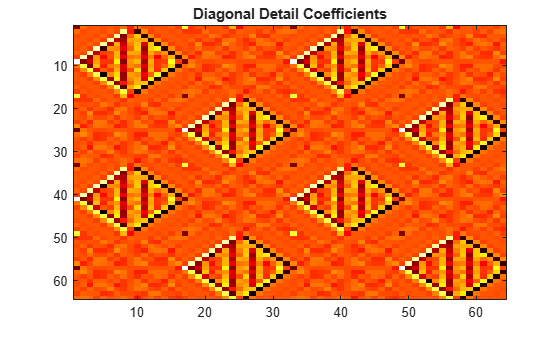imagesc(cA)
title('Approximation Coefficients')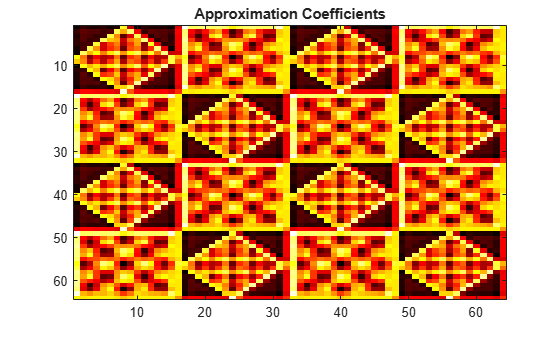imagesc(X)
colormap grayGenerate the lowpass and highpass decomposition filters for the Haar wavelet.

[LoD,HiD] = wfilters('haar','d');

Use the filters to perform a single-level 2-D wavelet decomposition. Use half-point symmetric extension. Display the approximation and detail coefficients.

[cA,cH,cV,cD] = dwt2(X,LoD,HiD,'mode','symh');
subplot(2,2,1)
imagesc(cA)
colormap gray
title('Approximation')
subplot(2,2,2)
imagesc(cH)
colormap gray
title('Horizontal')
subplot(2,2,3)
imagesc(cV)
colormap gray
title('Vertical')
subplot(2,2,4)
imagesc(cD)
colormap gray
title('Diagonal')Refer to GPU Support by Release (Parallel Computing Toolbox) to see what GPUs are supported.

Load an image. Put the image on the GPU using gpuArray. Save the current extension mode.

imgg = gpuArray(X);
origMode = dwtmode('status','nodisp');

Use dwtmode to change the extension mode to zero-padding. Obtain the single-level 2-D DWT of the image on the GPU using the db2 wavelet.

dwtmode('zpd','nodisp')
[cA,cH,cV,cD] = dwt2(imgg,'db2');

The current extension mode zpd is not supported for gpuArray input. Therefore, the DWT is instead performed using the sym extension mode. Confirm this by taking the DWT of imgg with the extension mode set to sym and compare with the previous result.

[cAsym,cHsym,cVsym,cDsym] = dwt2(imgg,'db2','mode','sym');
[max(abs(cA(:)-cAsym(:))) max(abs(cH(:)-cHsym(:))) ...
max(abs(cV(:)-cVsym(:))) max(abs(cD(:)-cDsym(:)))]
ans =

0     0     0     0

An unsupported extension mode specified as an input argument is converted to 'sym'. Confirm that taking the DWT of imgg with 'mode' set to an unsupported mode also defaults to the sym extension mode.

[cA,cH,cV,cD] = dwt2(imgg,'db2','mode','spd');
[max(abs(cA(:)-cAsym(:))) max(abs(cH(:)-cHsym(:))) ...
max(abs(cV(:)-cVsym(:))) max(abs(cD(:)-cDsym(:)))]
ans =

0     0     0     0

Change the current extension mode to periodic. Obtain the single-level DWT of the image on the GPU using the db2 wavelet.

dwtmode('per','nodisp')
[cA,cH,cV,cD] = dwt2(imgg,'db2');

Confirm the current extension mode per is supported for gpuArray input.

[cAper,cHper,cVper,cDper] = dwt2(imgg,'db2','mode','per');
[max(abs(cA(:)-cAper(:))) max(abs(cH(:)-cHper(:))) ...
max(abs(cV(:)-cVper(:))) max(abs(cD(:)-cDper(:)))]
ans =

0     0     0     0

Restore the extension mode to the original setting.

dwtmode(origMode,'nodisp')

Input Arguments

collapse all

Input data, specified as a numeric or logical array. X can be an m-by-n array representing an indexed image or an m-by-n-by-3 array representing a truecolor image. For more information on truecolor images, see RGB (Truecolor) Images.

Data Types: single | double | int8 | int16 | int32 | int64 | uint8 | uint16 | uint32 | uint64 | logical

Analyzing wavelet used to compute the 2-D DWT, specified as a character vector or string scalar. The analyzing wavelet is from one of the following wavelet families: Daubechies, Coiflets, Symlets, Fejér-Korovkin, Discrete Meyer, Biorthogonal, and Reverse Biorthogonal. See wfilters for the wavelets available in each family.

Wavelet decomposition lowpass filter, specified as an even-length real-valued vector. LoD must be of the same length as HiD.

Data Types: double | single

Wavelet decomposition highpass filter, specified as an even-length real-valued vector. HiD must be of the same length as LoD.

Data Types: double | single

Extension mode used when performing the DWT, specified as one of the following:

mode

DWT Extension Mode

'zpd'

Zero extension

'sp0'

Smooth extension of order 0

'spd' (or 'sp1')

Smooth extension of order 1

'sym' or 'symh'

Symmetric extension (half point): boundary value symmetric replication

'symw'

Symmetric extension (whole point): boundary value symmetric replication

'asym' or 'asymh'

Antisymmetric extension (half point): boundary value antisymmetric replication

'asymw'

Antisymmetric extension (whole point): boundary value antisymmetric replication

'ppd'

Periodized extension (1)

'per'

Periodized extension (2)

If the signal length is odd, wextend adds to the right an extra sample that is equal to the last value, and performs the extension using the 'ppd' mode. Otherwise, 'per' reduces to 'ppd'. This rule also applies to images.

The global variable managed by dwtmode specifies the default extension mode.

Example: [cA,cH,cV,cD] = dwt2(x,'db4','mode','symw');

Output Arguments

collapse all

Approximation coefficients, returned as an array whose size depends on X. Let sx = size(X) and lf = the length of the decomposition filters.

• If the DWT extension mode is set to periodization, then this output is of size ceil(sx/2).

• For the other extension modes, this output is of size floor((sx+lf-1)/2).

Data Types: double | single

Horizontal detail coefficients, returned as an array whose size depends on X. Let sx = size(X) and lf = the length of the decomposition filters.

• If the DWT extension mode is set to periodization, then this output is of size ceil(sx/2).

• For the other extension modes, this output is of size floor((sx+lf-1)/2).

Data Types: double | single

Vertical detail coefficients, returned as an array whose size depends on X. Let sx = size(X) and lf = the length of the decomposition filters.

• If the DWT extension mode is set to periodization, then this output is of size ceil(sx/2).

• For the other extension modes, this output is of size floor((sx+lf-1)/2).

Data Types: double | single

Diagonal detail coefficients, returned as an array whose size depends on X. Let sx = size(X) and lf = the length of the decomposition filters.

• If the DWT extension mode is set to periodization, then this output is of size ceil(sx/2).

• For the other extension modes, this output is of size floor((sx+lf-1)/2).

Data Types: double | single

Algorithms

The 2-D wavelet decomposition algorithm for images is similar to the one-dimensional case. The two-dimensional wavelet and scaling functions are obtained by taking the tensor products of the one-dimensional wavelet and scaling functions. This kind of two-dimensional DWT leads to a decomposition of approximation coefficients at level j in four components: the approximation at level j + 1, and the details in three orientations (horizontal, vertical, and diagonal). The following chart describes the basic decomposition steps for images.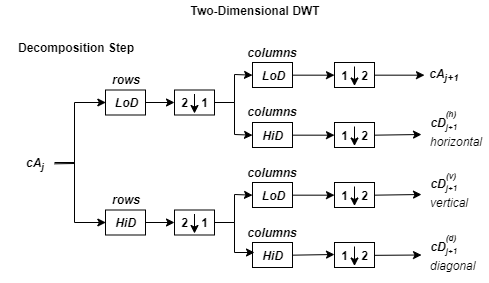where

•— Downsample columns: keep the even-indexed columns

•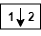— Downsample rows: keep the even-indexed rows

•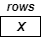— Convolve with filter X the rows of the entry

•— Convolve with filter X the columns of the entry

The decomposition is initialized by setting the approximation coefficients equal to the image s: cA0 = s.

Note

To deal with signal-end effects introduced by a convolution-based algorithm, the 1-D and 2-D DWT use a global variable managed by dwtmode. This variable defines the kind of signal extension mode used. The possible options include zero-padding and symmetric extension, which is the default mode.

 Daubechies, Ingrid. Ten Lectures on Wavelets. CBMS-NSF Regional Conference Series in Applied Mathematics 61. Philadelphia, Pa: Society for Industrial and Applied Mathematics, 1992.

 Mallat, S.G. “A Theory for Multiresolution Signal Decomposition: The Wavelet Representation.” IEEE Transactions on Pattern Analysis and Machine Intelligence 11, no. 7 (July 1989): 674–93. https://doi.org/10.1109/34.192463.

 Meyer, Y. Wavelets and Operators. Translated by D. H. Salinger. Cambridge, UK: Cambridge University Press, 1995.

Wavelet Toolbox DocumentationGet trial now